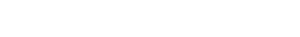Drag

# R统计代写｜Unit 2 Paper Appendices, Version 1

## Option A: GMP and population size

Prepare and submit four technical appendices, jointly produced from a single Rmd file. Your document

should include sections I-IV, as indicated below.

## Appendix I: Detail of statistical models2. Data for the project are at http://dept.stat.lsa.umich.edu/~bbh/s485/data/gmp-2006.csv
(http://dept.stat.lsa.umich.edu/~bbh/s485/data/gmp-2006.csv) . The fields are MSA name ( MSA ), per
capita GMP ( pcgmp ), and population ( pop ), as well as the shares of its economy deriving from:

finance; professional and technical services ( prof.tech ); information, communication and technology
( ict ); and management of firms and enterprises ( management ). Formulate two or three hypotheses
about how these other variables might influence per-capita GMP in a way that would produce the
appearance of supra-linear scaling when the additional variables were not properly taken into
account, while this appearance would disappear if those variables were properly accounted for. Write
your answer in words. (Hint: we’re asking you to report on an act of your imagination. There are no
right or wrong answers to this question, only more or less interesting answers, and more or less

## Appendix II: Exploratory analyses

1. Read in the data. Use the variables present in the data set to create a new variable representing the
GMP of the MSA: i.e. overall GMP, not per-capita GMP, and and other variables that may be
necessary to investigate hypotheses you formulated in (2) above.

2. Create scatter plots of GMP (Y) vs population size (x), of log GMP vs population size, of GMP vs
logged population size and of log GMP vs log population size. (This is overall GMP, not per-capita
GMP.) Add smoothed curves to each plot, without accompanying standard error envelopes. Which
is the better scale for capturing patterns in the data using a regression model of relatively simple
structure?

3. Starting with your preferred plot from (II.3) above, use colors, plotting symbols, etc to represent
differences between MSAs along whichever of finance , prof.tech , ict , management and any
constructed variables may be relevant to your hypotheses. (With ggplot2 this is done by adding
color, shape or other “aesthetics”, as described in the ggplot2 development team’s Web
documentation (http://ggplot2.tidyverse.org/reference/geom_point.html)

## Appendix III: Fitting the power law model

1. Use lm() to linearly regress log GMP – or the log of per-capita GMP, whichever is best – on the log
of population size. Explain how the estimates you get from this model can be translated into
estimates of the c and b in the power law scaling formula. Are these findings compatible with the
supra-linear power-law scaling hypothesis?

2. Plot your data so as to shed light on whether your model has captured the regression of of Y on X,
and on whether the errors/residuals have equal variances. Should we believe the standard errors that
the summary() function in R provides for the estimated coefficients of your model at the last step?

3. Using squared-error loss on the log scale, as the loss function,
calculate the in-sample loss, evaluated at estimated values of the parameters. (Hints: (a) Here
stands for the predicted value of the [log-ed] dependent variable, based on independent
variable N and parameter theta. (b) This is the same loss that lm() is implicitly minimizing when you
apply it to the logged variables.)